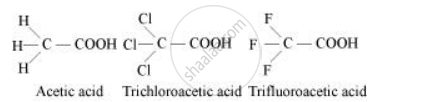Share

# The depression in freezing point of water observed for the same amount of acetic acid, trichloroacetic acid and trifluoroacetic acid increases in the order given above. Explain briefly. - CBSE (Science) Class 12 - Chemistry

ConceptColligative Properties and Determination of Molar Mass Depression of Freezing Point

#### Question

The depression in freezing point of water observed for the same amount of acetic acid, trichloroacetic acid and trifluoroacetic acid increases in the order given above. Explain briefly.

#### SolutionAmong H, Cl, and F, H is least electronegative while F is most electronegative. Then, F can withdraw electrons towards itself more than Cl and H. Thus, trifluoroacetic acid can easily lose H+ ions i.e., trifluoroacetic acid ionizes to the largest extent. Now, the more ions produced, the greater is the depression of the freezing point. Hence, the depression in the freezing point increases in the order:

Acetic acid < trichloroacetic acid < trifluoroacetic acid

Is there an error in this question or solution?

#### Video TutorialsVIEW ALL 

Solution The depression in freezing point of water observed for the same amount of acetic acid, trichloroacetic acid and trifluoroacetic acid increases in the order given above. Explain briefly. Concept: Colligative Properties and Determination of Molar Mass - Depression of Freezing Point.
S